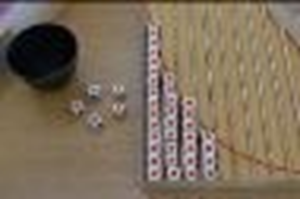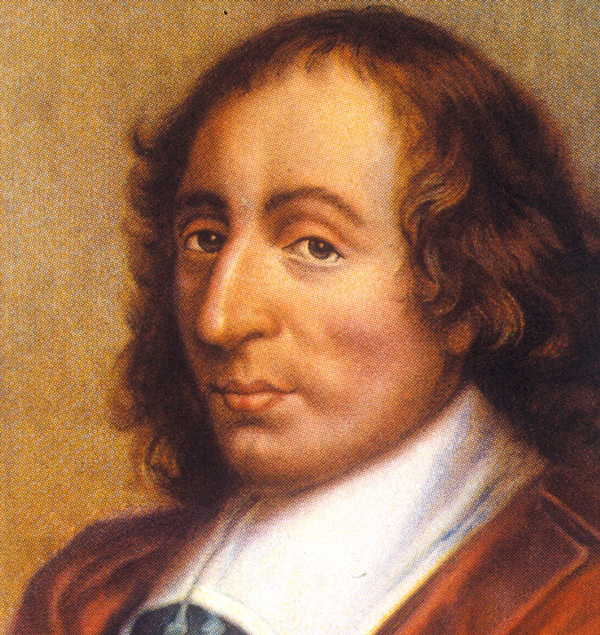# Red Dice OutRoll all dice and put the red dice in the first column. Does it follow the prediction? Repeat with the remaining dice, do the dice follow the curve? What proportion of dice will be red each time? If I start with 100 dice, how many blue dice will be left after five rolls? If I have 20 blue dice left after ten rolls, how many dice did I start with?
Maths

Each die has two red sides and four blue sides. So, every time we roll we expect 2/6 (one-third) of dice to be red, and 4/6 (two-thirds) of dice to be blue.
Each time we roll, two-thirds of the dice will be blue, and so the number of blue dice will get smaller with each roll. This is called exponential decay. After rolling n times, (2/3)n of the original dice are blue.
Each roll may not happen exactly as predicted, but it is unlikely to be hugely different. Although we cannot predict what will happen to each die individually, we can make general predictions for large number of dice.

History

In 1654, French writer Antoine Gombaud imagined a gambling game for two players. Then he asked, if the game is interrupted, how should the money be divided? For example, if the first player is winning 7 rounds to 4 (with 4 round left to play), should all the money go to the player with the most points? Or should the money be divided in proportion to the number of rounds already won?
Mathematicians Blaise Pascal and Pierre de Fermat took a different approach. They considered what would have happen if the game had continued, and divided the money based on how many of these possible futures were won by each player. For the first time, Pascal and Fermat were using mathematics to predict the future.

PeoplePierre de Fermat 1607 – 1665 Probably the greatest amateur mathematician in history. He was a lawyer who also corresponded with the leading mathematicians of the day. In this way he made fundamental contributions to many branches of mathematics including geometry, number theory, the development of calculus, and probability. Fermat’s last theorem is famous because he didn’t have room to write out his proof of it and it wasn’t until 1995 that Andrew Wiles finally proved it. Blaise Pascal 1623 – 1662 He was a philosopher and mathematician. His correspondence with Fermat to solve a problem of calculating the fair division of money in an incomplete betting game is now seen as the foundation of the modern theory of probability theory. After a religious conversion Pascal gave up mathematics for many years. He also analysed the pattern of numbers of binomial coefficients which is now known in the west as Pascal’s triangle.Applications

Exponential decay is found in many natural processes. For example, radioactive material decays exponentially. The half-life of a radioactive element is the time it takes for half the material to decay.
Another example is atmospheric pressure, which decreases by 12% for every 1000m height above sea level.

On the other hand, exponential growth is when something grows, with a rate greater than 1. For example, with enough food, bacteria will split into two cells, which then split into four cells and so on, doubling the amount of bacteria with each division.
Another example of exponential growth is compound interest, such as a bank account that offers 2% interest will grow by that amount each year.

Maths at Home

Start with four dice. Roll the dice and count the number of dice with even numbers. Add a new die for every die showing an even number, and roll again. Repeat the procedure at least five more times. How many dice do you have after each roll? What is the growth rate? How many times would I need to roll to have 100 dice?
If you don’t have dice, you could use coins, or sweets like M&Ms or skittles (using letter-side-up and letter-side-down).

Here is a famous riddle about exponential growth:
A water lilly is growing in a pond and doubles in size every day. If left alone, the water lilly will cover the entire pond in 30 days – killing all the other living things in the water. Day after day, the plant’s growth is small, so it is decided that it won’t be a concern until it covers half of the pond. Which day will that be?